### Afternoondragon Step03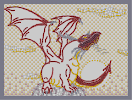Hover over the thumbnail for a full-size version.

Author Dragon_Moon author:dragon_moon n-art rated 2006-03-07 4 by 41 people. \$Afternoondragon Step03#Dragon_Moon#none#00000000000000000000000000000000000000000000000000000000000000000000000000000000000000000000000000000000000000000000000000000000000000000000000000000000000000000000000000000000000000000000000000000000000000000000000000000000000000000000000000000000000000000000000000000000000000000000000000000000000000000000000000000000000000000000000000000000000000000000000000000000000000000000000000000000000000000000000000000000000000000000000000000000000000000000000000000000000000000000000000000000000000000000000000000000000000000000000000000000000000000000000000000000000000000000000000000000000000000000000000000000000000000000000000000000000000000000000000000000000000000000000000000000000000000000000000000000000000000|5^598,206!12^660,414!12^605,203!12^601,203!12^595,203!12^589,203!12^578,205!12^572,208!12^585,205!12^575,207!12^568,211!12^566,213!12^562,216!12^558,221!12^553,225!12^550,228!12^546,232!12^542,235!12^535,240!12^525,244!12^520,247!12^514,250!12^507,254!12^503,256!12^501,261!12^495,266!12^492,266!12^498,265!12^540,237!12^522,246!12^531,242!12^581,204!12^511,252!12^517,249!12^604,200!12^600,197!12^594,194!12^590,194!12^587,193!12^582,192!12^575,192!12^569,192!12^553,201!12^549,204!12^544,208!12^538,210!12^532,210!12^526,210!12^523,210!12^517,210!12^511,211!12^504,212!12^499,213!12^494,217!12^490,219!12^485,223!12^483,226!12^477,232!12^479,228!12^475,233!12^472,236!12^468,238!12^461,240!12^454,242!12^457,241!12^465,239!12^500,230!12^504,230!12^504,228!12^508,228!12^512,228!12^525,220!12^522,223!12^520,227!12^520,223!12^516,224!12^512,224!12^511,224!12^508,224!12^519,225!12^504,226!0^505,227!0^510,226!0^515,225!12^594,210!12^594,212!12^593,216!12^593,220!12^593,223!12^592,228!12^591,230!12^590,233!12^588,236!12^587,240!12^586,245!12^583,247!12^581,250!12^578,254!12^577,258!12^575,261!12^572,262!12^566,264!12^559,266!12^553,266!12^547,270!12^544,275!12^540,280!12^537,284!12^532,289!12^529,294!12^526,298!12^521,301!12^471,282!12^515,300!12^509,298!12^505,300!12^500,297!12^495,295!12^488,295!12^487,290!12^482,287!12^475,287!12^478,287!12^472,285!12^492,296!12^500,299!7^593,253,2!7^570,261,2!7^581,259,2!7^573,275,2!7^596,277,2!7^576,282,1!7^581,272,1!7^588,298,1!7^600,292,1!7^613,313,1!7^625,305,1!7^643,304,1!7^627,287,1!7^597,280,1!7^583,261,1!7^553,267,1!7^572,241,1!2^529,202,-0.707106781186547,-0.707106781186547!2^538,192,-1,0!2^535,205,-0.707106781186547,-0.707106781186547!2^514,309,-0.707106781186547,-0.707106781186547!2^507,319,-0.707106781186547,-0.707106781186547!2^508,308,-0.707106781186547,-0.707106781186547!2^501,318,-0.707106781186547,-0.707106781186547!2^498,329,-0.707106781186547,-0.707106781186547!2^492,336,-1,0!2^492,333,-0.707106781186547,-0.707106781186547!2^488,346,-0.707106781186547,-0.707106781186547!2^487,352,-1,0!2^524,207,0,-1!12^449,242!12^444,243!12^441,245!12^438,245!12^434,247!12^430,248!12^429,248!12^427,251!12^424,252!12^422,252!12^420,254!12^417,256!12^414,258!12^411,258!12^408,260!12^407,261!12^405,263!12^403,264!12^401,266!12^399,267!12^396,270!12^395,272!12^392,275!12^390,278!12^387,282!12^384,285!12^382,288!12^380,293!12^378,296!12^375,302!12^376,301!12^375,308!12^375,312!12^375,319!12^375,306!12^376,317!12^376,324!12^376,328!12^376,333!12^376,337!12^377,341!12^378,341!12^378,345!12^378,348!12^378,352!12^378,357!12^377,361!12^376,363!12^375,366!2^458,239,0,-1!2^446,237,0,-1!2^433,234,0,-1!2^423,230,0,-1!2^411,228,0.707106781186547,-0.707106781186547!2^403,223,0,-1!2^392,222,0.707106781186547,-0.707106781186547!2^387,218,0,-1!2^378,218,0.707106781186547,-0.707106781186547!2^370,214,0,-1!2^365,210,0.707106781186547,-0.707106781186547!2^357,204,0.707106781186547,-0.707106781186547!2^355,196,0.707106781186547,-0.707106781186547!2^364,217,0.707106781186547,-0.707106781186547!2^373,222,0.707106781186547,-0.707106781186547!2^388,226,0.707106781186547,-0.707106781186547!2^387,229,0,-1!2^403,230,0.707106781186547,-0.707106781186547!2^399,230,0,-1!2^417,239,0.707106781186547,-0.707106781186547!2^428,240,0,-1!2^431,242,-0.707106781186547,-0.707106781186547!2^440,240,0,-1!2^420,235,0,-1!2^394,206,0.707106781186547,-0.707106781186547!2^408,214,0.707106781186547,-0.707106781186547!2^403,215,0.707106781186547,-0.707106781186547!2^416,220,0.707106781186547,-0.707106781186547!2^428,223,0,-1!2^436,228,0.707106781186547,-0.707106781186547!2^450,234,0,-1!2^393,200,0.707106781186547,-0.707106781186547!2^496,308,-0.707106781186547,-0.707106781186547!2^490,304,-0.707106781186547,-0.707106781186547!2^483,316,-0.707106781186547,-0.707106781186547!2^488,313,-0.707106781186547,-0.707106781186547!2^477,323,-0.707106781186547,-0.707106781186547!2^476,330,-1,0!2^472,338,-0.707106781186547,-0.707106781186547!2^468,345,-1,0!2^472,334,-1,0!2^483,298,-0.707106781186547,-0.707106781186547!2^474,304,-0.707106781186547,-0.707106781186547!2^465,309,-1,0!2^462,317,-0.707106781186547,-0.707106781186547!2^457,323,-1,0!2^455,334,-0.707106781186547,-0.707106781186547!2^454,328,-1,0!2^450,337,-1,0!2^459,311,-0.707106781186547,-0.707106781186547!2^469,303,-0.707106781186547,-0.707106781186547!2^478,297,-0.707106781186547,-0.707106781186547!2^467,289,0,-1!2^457,291,0,-1!2^451,295,0,-1!2^444,301,-0.707106781186547,-0.707106781186547!2^438,309,0,-1!2^434,313,-1,0!2^438,312,-0.707106781186547,-0.707106781186547!2^446,301,-0.707106781186547,-0.707106781186547!2^455,298,-0.707106781186547,-0.707106781186547!2^462,293,0,-1!2^471,292,0,-1!12^558,197!12^564,194!12^578,192!12^598,196!12^562,193!0^480,306!0^475,311!0^470,315!0^465,320!0^462,326!0^460,332!0^457,340!0^458,346!0^460,352!0^462,359!0^467,373!0^465,367!0^464,363!0^458,336!12^472,278!12^492,293!12^497,290!12^507,297!12^509,293!12^479,282!0^471,380!0^470,378!0^473,387!0^470,391!0^471,396!0^473,402!0^473,408!0^471,415!0^467,415!0^467,422!0^467,428!0^466,435!0^465,439!0^464,445!0^460,445!0^465,392!0^462,395!0^456,396!0^451,395!0^446,392!0^457,352!0^452,353!0^447,353!0^443,352!0^440,346!0^438,341!0^441,390!0^437,387!0^435,383!0^435,379!0^459,446!0^455,445!0^452,445!0^448,444!0^443,443!0^439,441!0^433,435!0^431,431!0^428,424!0^426,419!0^437,439!0^428,415!12^402,389!12^407,390!12^409,393!12^410,393!12^410,397!12^414,400!12^418,406!12^420,411!12^421,416!12^421,424!12^421,430!12^421,420!12^420,435!12^420,441!12^419,443!12^417,448!12^417,453!12^416,458!12^415,463!12^412,465!12^408,471!12^408,473!12^408,476!12^409,470!12^401,416!12^396,427!12^396,432!12^396,437!12^396,443!12^396,447!12^399,457!12^409,481!12^410,485!12^412,490!12^414,497!12^416,504!12^420,509!12^416,512!12^423,513!12^427,516!12^430,519!12^435,523!12^440,526!12^444,530!12^449,534!12^452,538!12^452,544!12^451,546!12^427,533!12^433,535!12^436,538!12^439,543!12^441,549!12^423,531!12^418,525!12^442,524!12^447,524!12^453,526!12^459,530!12^462,534!12^465,539!12^426,558!12^423,553!12^423,549!12^425,546!12^420,549!12^417,549!12^413,546!12^376,517!12^373,514!12^372,512!12^375,499!12^375,492!12^373,509!12^374,505!12^376,497!12^374,488!12^374,484!12^369,478!12^368,475!12^365,472!12^362,465!12^360,460!12^360,456!12^361,446!0^458,451!0^458,458!0^457,464!0^454,470!0^450,475!0^445,481!0^441,487!0^435,491!0^430,488!0^424,485!0^419,481!0^415,479!0^413,476!0^430,493!0^428,498!0^424,504!0^425,505!0^427,501!12^361,450!12^398,422!12^399,452!12^374,368!12^372,368!12^371,370!12^370,370!12^350,375!12^346,373!12^343,372!12^338,370!12^336,370!12^331,370!12^328,369!12^325,369!12^319,369!12^314,369!12^309,369!12^296,372!12^293,374!12^269,388!12^265,391!12^263,393!12^252,415!12^251,420!12^250,429!12^305,369!12^300,370!12^289,376!12^286,378!12^282,380!12^277,383!12^272,385!12^261,396!12^258,400!12^256,404!12^254,409!12^250,424!12^248,432!12^357,378!12^372,359!12^368,355!12^366,354!12^363,351!12^357,349!12^355,348!12^350,344!12^346,341!12^343,339!12^339,335!12^336,332!12^332,329!12^329,325!12^326,322!12^323,318!12^319,312!12^315,308!12^311,303!12^308,298!12^304,293!12^302,289!12^300,287!12^294,281!12^290,279!12^286,277!12^282,277!12^280,277!12^296,284!12^282,274!12^282,274!12^285,270!12^289,267!12^292,262!12^293,261!12^299,221!12^297,214!12^296,213!12^294,208!12^295,257!12^298,253!12^300,247!12^300,242!12^301,239!12^301,237!12^301,234!12^301,232!12^300,229!12^300,226!12^299,224!12^297,216!12^294,205!12^294,203!12^293,199!12^293,196!12^293,193!12^293,190!12^293,188!12^293,185!12^293,181!12^293,176!12^294,173!12^294,170!12^294,166!12^294,161!12^294,157!12^299,151!12^295,154!12^305,148!12^310,150!12^316,147!12^317,143!12^312,131!12^308,131!12^304,131!12^299,132!12^291,132!12^294,134!12^286,128!12^270,117!12^251,105!12^230,91!12^203,77!12^281,125!12^278,122!12^275,120!12^266,113!12^262,111!12^255,108!12^257,109!12^248,103!12^246,102!12^243,100!12^240,97!12^238,96!12^236,95!12^234,93!12^231,91!12^228,90!12^224,87!12^219,84!12^215,82!12^212,80!12^206,77!12^203,76!12^200,74!12^198,73!12^195,71!12^192,70!12^190,68!12^187,67!12^181,65!12^176,62!12^171,60!12^166,58!12^161,56!12^157,55!12^154,54!12^148,51!12^147,51!12^142,49!12^137,48!12^135,48!12^127,46!12^122,45!12^118,44!12^112,43!12^108,42!12^102,41!12^96,41!12^89,41!12^84,41!12^79,42!12^69,43!12^67,43!12^63,43!12^55,44!12^51,45!12^45,45!12^58,44!12^91,41!12^130,47!12^73,42!12^151,53!12^59,148!12^297,370!12^299,367!12^299,363!12^299,357!12^296,352!12^292,349!12^290,346!12^287,342!12^132,235!12^132,232!12^132,231!12^133,226!12^134,221!12^135,215!12^135,210!12^135,204!12^135,200!12^131,193!12^129,190!12^125,184!12^120,178!12^116,172!12^114,169!12^111,167!12^107,164!12^103,160!12^97,156!12^93,154!12^89,153!12^85,151!12^80,150!12^75,150!12^68,150!12^64,150!12^61,150!12^56,150!12^54,151!12^53,151!12^52,152!12^53,151!12^58,145!12^60,144!12^64,138!12^65,134!12^67,129!12^67,124!12^67,118!12^68,113!12^68,106!12^68,101!12^68,96!12^62,80!12^58,75!12^55,72!12^46,65!12^44,63!12^43,62!12^37,58!12^33,56!12^32,56!12^32,56!12^34,52!12^36,50!12^42,47!12^45,45!12^28,55!12^50,68!12^39,61!12^64,84!12^67,88!12^68,92!12^61,141!12^48,154!12^119,176!12^123,182!12^134,200!12^127,187!12^132,197!12^99,157!12^295,349!12^289,344!12^285,339!12^282,336!12^278,331!12^276,326!12^274,323!12^271,320!12^268,315!12^266,311!12^264,306!12^261,302!12^260,297!12^258,293!12^257,291!12^254,284!12^250,282!12^249,282!12^245,279!12^245,276!12^246,271!12^250,268!12^251,263!12^252,259!12^255,253!12^255,252!12^255,245!12^256,240!12^256,235!12^258,230!12^259,225!12^260,220!12^260,216!12^256,247!12^255,288!12^260,213!12^260,211!12^262,201!12^264,196!12^265,192!12^261,208!12^267,187!12^267,186!12^268,179!12^268,173!12^270,166!12^272,162!12^275,156!12^278,153!12^280,148!12^279,149!12^272,149!12^266,149!12^264,149!12^258,149!12^253,149!12^252,150!12^247,150!12^243,150!12^237,150!12^233,150!12^226,151!12^220,153!12^210,157!12^206,157!12^201,160!12^197,161!12^187,167!12^182,169!12^178,172!12^174,175!12^170,179!12^157,190!12^152,195!12^148,198!12^143,205!12^138,213!12^135,218!12^125,241!12^127,238!12^146,201!12^141,209!12^153,192!12^160,187!12^165,183!12^191,164!12^215,154!12^217,151!12^219,151!12^220,151!12^224,148!12^225,147!12^230,146!12^232,146!12^235,145!12^237,145!12^240,144!12^242,144!12^245,143!12^249,143!12^253,143!12^256,143!12^259,142!12^263,142!12^265,142!12^267,142!12^270,142!12^273,142!12^276,142!12^277,142!12^219,147!12^217,149!12^213,152!12^212,152!12^208,155!12^208,156!12^207,154!12^204,155!12^200,156!12^195,159!12^188,163!12^193,162!12^184,168!12^274,147!12^270,139!12^264,137!12^259,135!12^257,133!12^253,130!12^249,128!12^246,126!12^243,125!12^237,122!12^232,120!12^228,119!12^221,118!12^216,117!12^209,116!12^202,116!12^123,117!12^116,119!12^108,120!12^102,122!12^96,125!12^93,126!12^84,132!12^81,134!12^79,135!12^74,139!12^69,141!12^66,142!12^64,142!12^61,145!12^71,141!12^98,123!12^104,122!12^104,122!12^112,120!12^128,118!12^119,118!12^200,116!12^87,128!12^90,128!12^272,136!12^269,136!12^265,132!12^263,130!12^261,130!12^258,127!12^255,124!12^251,123!12^249,123!12^246,123!12^245,123!12^243,122!12^240,119!12^237,118!12^234,117!12^231,116!12^225,115!12^223,114!12^219,114!12^215,114!12^210,114!12^206,114!12^129,116!12^225,119!12^204,114!12^202,114!12^198,114!12^242,122!12^133,116!12^140,115!12^141,114!12^145,114!12^148,114!12^150,113!12^154,113!12^158,113!12^161,113!12^166,113!12^172,113!12^176,113!12^185,113!12^193,113!12^198,114!12^192,113!12^184,112!12^181,113!12^187,115!12^191,115!12^197,116!12^194,116!12^181,115!12^180,114!12^177,114!12^173,114!12^168,114!12^164,114!12^159,114!12^137,116!12^76,137!12^37,51!12^281,131!12^279,129!12^271,122!12^268,121!12^267,120!12^261,115!12^283,135!12^277,131!12^274,129!12^270,128!12^266,125!12^263,120!12^262,119!12^260,117!12^258,116!12^256,115!12^254,113!12^252,112!12^251,111!12^249,110!12^246,108!12^246,106!12^244,105!12^243,104!12^241,102!12^239,101!12^236,100!12^234,98!12^232,97!12^230,96!12^228,94!12^225,92!12^223,90!12^219,88!12^217,87!12^215,85!12^212,83!12^210,82!12^207,80!12^204,78!12^200,77!12^197,75!12^192,72!12^187,70!12^182,68!12^181,67!12^178,67!12^175,64!12^172,64!12^170,61!12^168,60!12^166,59!12^162,58!12^157,57!12^152,55!12^150,54!12^146,53!12^141,50!12^142,52!12^136,49!12^274,124!12^286,133!12^278,137!12^274,135!12^271,133!12^265,128!12^261,124!12^281,225!12^279,228!12^277,232!12^275,235!12^273,238!12^273,242!12^273,250!12^273,252!12^273,247!12^283,297!12^283,303!12^285,311!12^287,315!12^293,321!12^295,322!12^284,308!12^291,321!12^298,324!12^301,326!12^305,330!12^310,333!12^314,337!12^318,339!12^290,319!12^283,220!12^134,235!12^136,235!12^143,233!12^144,233!12^147,232!12^151,232!12^156,232!12^164,233!12^169,236!12^175,237!12^182,238!12^197,246!12^199,247!12^200,247!12^204,250!12^208,252!12^215,256!12^225,267!12^227,268!12^252,295!12^258,305!12^263,312!12^265,316!12^268,321!12^270,328!12^271,332!12^276,345!12^276,346!12^276,350!12^276,356!12^276,361!12^273,379!12^270,384!12^275,375!12^275,370!12^276,365!12^276,365!12^274,342!12^273,339!12^273,337!12^268,324!12^254,298!12^249,292!12^246,288!12^242,284!12^240,283!12^238,280!12^236,279!12^234,277!12^233,274!12^230,272!12^228,271!12^227,270!12^226,268!12^224,265!12^222,263!12^220,262!12^217,259!12^215,256!12^211,253!12^209,252!12^206,249!12^203,247!12^195,245!12^193,243!12^190,241!12^187,240!12^185,239!12^183,238!12^180,238!12^177,236!12^174,236!12^169,234!12^165,233!12^160,232!12^155,231!12^140,234!12^440,553!12^372,482!12^363,471!12^418,445!2^432,554,1,0!2^435,562,1,0!2^433,567,0.707106781186547,0.707106781186547!2^427,560,1,0!2^432,561,1,0!2^431,557,1,0!2^429,568,0.707106781186547,0.707106781186547!2^445,549,0.707106781186547,-0.707106781186547!2^448,556,0.707106781186547,-0.707106781186547!2^452,554,0.707106781186547,-0.707106781186547!2^455,560,1,0!2^453,560,1,0!2^460,546,0.707106781186547,-0.707106781186547!2^462,542,0.707106781186547,-0.707106781186547!2^466,549,1,0!2^466,550,1,0!2^467,552,1,0!2^316,137,-0.707106781186547,-0.707106781186547!2^314,141,-0.707106781186547,-0.707106781186547!2^310,139,0,-1!2^311,140,0,-1!2^310,138,-0.707106781186547,-0.707106781186547!2^318,132,0,-1!2^327,133,0,-1!2^334,134,0.707106781186547,-0.707106781186547!2^320,136,0,-1!2^333,134,0.707106781186547,-0.707106781186547!2^331,134,0,-1!12^495,292!12^509,296!12^472,280!12^287,379!12^293,378!12^295,375!12^295,379!12^316,407!12^316,409!12^317,412!12^317,413!12^317,415!12^317,419!12^317,421!12^316,426!12^316,426!12^316,427!12^316,428!12^316,431!12^316,433!12^315,436!12^315,438!12^314,440!12^314,443!12^315,444!12^313,446!12^312,448!12^312,449!12^312,451!12^312,454!12^310,455!12^310,457!12^309,460!12^308,463!12^308,463!12^305,466!12^305,466!12^304,468!12^304,469!12^302,470!12^301,472!12^299,476!12^298,478!12^297,480!12^295,481!12^294,484!12^292,485!12^290,485!12^288,486!12^285,489!12^283,491!12^282,492!12^278,493!12^275,493!12^249,440!12^249,446!12^248,435!12^250,451!12^251,453!12^251,454!12^251,456!12^251,457!12^253,461!12^253,464!12^254,467!12^255,470!12^255,472!12^256,477!12^257,479!12^258,482!12^260,486!12^260,487!12^263,488!12^265,490!12^266,491!12^267,493!12^269,493!12^273,495!12^288,490!12^288,489!12^306,465!12^249,439!12^275,494!12^262,491!12^295,491!12^295,491!12^295,495!12^296,499!12^298,503!12^299,508!12^301,510!12^301,511!12^304,516!12^305,522!12^307,523!12^264,496!12^263,500!12^263,505!12^262,507!12^261,510!12^261,511!12^260,515!12^259,517!12^258,519!12^257,523!12^255,525!12^255,528!12^255,529!12^308,528!12^308,528!12^266,535!12^264,532!12^261,530!12^259,530!12^256,530!12^253,532!12^252,538!12^252,543!12^254,545!12^255,550!12^255,553!12^267,539!12^269,541!12^269,544!12^269,547!12^269,552!12^273,536!12^273,535!12^275,532!12^275,530!12^278,527!12^280,526!12^283,523!12^285,522!12^289,525!12^290,531!12^291,534!12^291,539!12^291,544!12^291,549!12^291,552!12^288,555!12^288,556!12^287,560!12^271,555!12^274,560!12^267,556!12^298,534!12^301,532!12^302,531!12^304,530!12^307,530!12^313,532!12^313,538!12^313,542!12^313,546!12^311,549!12^310,552!12^309,552!12^309,554!12^296,540!12^297,544!12^296,548!12^296,558!12^295,554!12^412,547!12^373,517!12^371,513!12^371,506!12^423,552!12^420,529!12^430,534!12^448,532!12^422,511!12^418,511!12^418,507!12^415,500!12^413,493!12^411,490!12^408,484!12^405,477!12^453,525!12^452,525!12^444,523!12^438,522!12^434,522!12^410,547!12^409,547!12^407,546!12^404,546!12^402,546!12^402,546!12^398,544!12^396,543!12^395,543!12^395,539!12^393,536!12^391,533!12^389,529!12^387,527!12^382,523!12^380,522!12^376,520!12^387,527!2^388,538,-0.707106781186547,0.707106781186547!2^385,536,-1,0!2^381,541,-1,0!2^260,556,-1,0!2^263,561,-1,0!2^261,566,-1,0!2^278,555,-1,0!2^280,555,1,0!2^275,556,1,0!2^277,553,1,0!2^277,563,1,0!2^277,570,1,0!2^300,555,1,0!2^304,550,1,0!2^304,559,1,0!2^306,560,1,0!2^307,568,1,0!0^334,509!0^356,518!0^362,522!0^369,524!0^311,500!0^307,500!0^350,518!0^343,519!0^337,518!0^337,513!0^339,510!0^344,507!0^349,502!0^351,499!0^354,494!0^354,487!0^354,481!0^330,511!0^323,510!0^318,508!0^314,508!0^318,504!0^321,499!0^325,494!0^327,490!0^327,481!0^326,486!0^324,475!0^303,500!0^307,492!0^310,488!0^310,486!0^310,480!0^305,496!0^309,475!0^309,473!0^252,482!0^249,477!0^245,468!0^243,462!0^242,454!0^242,443!0^242,450!0^244,460!0^248,473!0^245,465!0^243,440!0^245,437!12^247,438!0^256,487!12^260,486!0^245,432!0^246,427!0^243,437!12^247,437!12^249,432!12^249,425!12^464,454!12^469,457!12^473,462!12^480,471!12^480,471!12^484,472!12^488,476!12^492,478!12^496,480!12^500,482!12^503,483!12^507,484!12^510,485!12^513,486!12^517,487!12^522,487!12^526,487!12^530,487!12^535,487!12^539,487!12^542,487!12^545,487!12^550,487!12^556,486!12^560,484!12^563,483!12^569,481!12^572,480!12^574,479!12^579,478!12^582,476!12^584,473!12^589,470!12^592,468!12^594,467!12^596,466!12^597,466!12^598,463!12^600,462!12^600,461!12^602,459!12^605,457!12^607,455!12^609,453!12^611,451!12^612,450!12^613,449!12^615,448!12^617,446!12^617,445!12^619,444!12^620,443!12^622,441!12^623,441!12^623,440!12^626,437!12^627,437!12^628,435!12^630,433!12^632,431!12^633,430!12^634,429!12^635,428!12^637,426!12^638,425!12^587,472!12^581,476!12^578,478!12^576,479!12^571,480!12^568,480!12^565,481!12^563,481!12^560,483!12^556,483!12^550,485!12^486,475!12^483,474!12^480,471!12^479,469!12^477,466!12^475,464!12^472,461!12^471,460!12^470,460!12^468,458!12^466,457!12^465,452!0^459,454!0^463,448!12^631,413!12^633,412!12^628,415!12^624,417!12^624,417!12^621,420!12^620,422!12^622,417!12^627,414!12^630,412!12^633,409!12^642,400!12^647,395!12^651,391!12^654,387!12^658,382!12^659,383!12^659,386!12^658,381!12^660,379!12^661,378!12^661,377!12^663,373!12^663,370!12^665,367!12^665,365!12^662,380!12^661,383!12^661,386!12^661,388!12^661,391!12^661,395!12^661,399!12^661,403!12^662,409!12^662,413!12^662,418!12^663,426!12^663,429!12^664,434!12^665,434!12^663,423!12^663,435!12^663,432!12^662,430!12^660,429!12^658,429!12^655,429!12^653,429!12^652,429!12^645,439!12^643,441!12^642,444!12^641,446!12^640,449!12^638,451!12^636,453!12^634,456!12^632,459!12^630,462!12^629,464!12^627,466!12^626,468!12^623,470!12^620,474!12^619,477!12^615,482!12^610,487!12^603,494!12^647,437!12^665,439!12^645,398!12^638,417!12^636,415!12^635,415!12^639,422!12^648,432!12^637,405!12^640,402!0^439,497!0^445,502!0^452,506!0^457,510!0^471,516!0^477,517!0^486,520!0^492,520!0^503,521!0^511,521!0^521,521!0^531,521!0^539,521!0^550,521!0^562,519!0^572,517!0^581,512!0^587,508!0^591,504!0^595,502!0^599,499!0^601,498!0^607,495!0^609,490!0^614,485!0^612,487!0^617,482!0^451,485!0^456,490!0^462,493!0^470,499!0^480,504!0^490,507!0^505,509!0^518,511!0^529,513!0^581,509!0^588,507!0^443,500!0^436,495!0^450,505!0^450,483!0^455,489!0^460,493!0^466,497!0^476,503!0^474,501!0^485,506!0^494,508!0^500,509!0^509,510!0^513,510!0^524,512!0^535,514!0^540,515!0^545,516!0^550,516!0^555,517!0^561,517!0^568,516!0^576,512!0^572,514!0^578,514!0^556,520!0^567,518!0^463,513!0^467,515!0^482,519!0^499,521!0^508,522!0^517,521!0^528,521!0^545,522!0^457,493!0^454,498!0^451,499!0^477,507!0^474,511!0^470,513!0^499,513!0^492,518!0^489,519!0^518,517!12^616,480!12^611,485!12^608,489!12^604,493!12^600,496!12^594,499!12^588,501!12^583,503!12^456,480!12^459,485!12^462,489!12^467,492!12^470,495!12^475,498!12^479,500!12^536,512!12^542,513!12^545,513!12^548,513!12^552,513!12^555,513!12^558,513!12^561,513!12^566,511!12^570,510!12^574,507!12^578,505!12^453,477!0^449,478!0^451,474!12^481,502!12^486,504!12^490,505!12^494,506!12^500,507!12^505,507!12^511,508!12^513,509!12^518,510!12^530,512!12^524,511!0^600,528!0^504,396!0^612,372!0^528,528!0^648,480!0^490,418!0^526,376!0^585,364!0^633,391!0^660,453!0^625,509!0^484,464!0^563,533!0^482,455!0^485,431!0^482,442!0^497,406!0^515,385!0^538,367!0^569,362!0^554,363!0^599,368!0^626,381!0^658,440!0^656,468!0^638,494!0^615,520!0^583,533!0^546,532!12^404,249!12^340,195!12^403,244!12^423,206!12^427,203!12^432,198!12^435,196!12^437,195!12^438,193!12^440,190!12^442,188!12^444,185!12^446,182!12^447,180!12^449,175!12^451,173!12^453,168!12^455,163!12^455,160!12^455,155!12^457,150!12^459,146!12^461,137!12^465,131!12^468,128!12^470,124!12^459,141!12^462,135!12^429,202!2^411,213,0.707106781186547,-0.707106781186547!12^456,151!12^457,148!12^454,166!12^452,170!12^448,178!12^472,126!12^477,133!12^477,131!12^478,109!12^483,112!12^484,115!12^484,119!12^484,120!12^476,106!12^474,105!12^475,106!12^468,104!12^462,102!12^459,102!12^456,101!12^450,98!12^444,97!12^437,96!12^431,96!12^425,96!12^417,96!12^410,96!12^401,96!12^394,96!12^386,96!12^380,96!12^373,98!12^364,100!12^357,100!12^349,102!12^342,103!12^338,105!12^332,107!12^326,107!12^315,112!12^307,115!12^321,109!12^370,99!12^318,113!12^324,112!12^333,111!12^339,112!12^344,112!12^352,117!12^355,120!12^356,130!12^356,135!12^355,144!12^350,151!12^347,157!12^343,162!12^340,167!12^328,180!12^325,183!12^337,170!12^322,189!12^329,191!12^340,196!12^345,199!12^347,205!12^347,232!12^344,238!12^345,245!12^350,245!12^353,336!12^351,339!12^356,331!12^358,326!12^360,320!12^361,313!12^362,304!12^361,308!12^353,248!12^356,251!12^360,255!12^364,258!12^365,261!12^367,264!12^368,268!12^367,274!12^366,278!12^366,282!12^365,287!12^364,291!12^363,297!12^364,300!12^404,253!12^403,258!12^349,228!12^349,220!12^349,213!12^349,209!12^334,192!12^333,175!12^356,123!12^357,127!12^347,113!12^327,111!12^311,113!12^357,139!12^353,147!12^349,224!12^335,104!12^354,101!12^376,97!12^390,97!12^407,95!12^440,97!12^414,96!12^398,96!12^360,99!12^453,100!2^478,123,0.707106781186547,-0.707106781186547!2^485,130,0.707106781186547,-0.707106781186547!2^488,136,0.707106781186547,-0.707106781186547!2^490,141,1,0!2^487,137,0.707106781186547,-0.707106781186547!2^477,128,0.707106781186547,-0.707106781186547!2^476,122,0.707106781186547,-0.707106781186547!2^477,120,1,0!12^326,186!12^469,112!12^472,111!12^463,112!12^458,110!12^452,109!12^448,109!12^443,109!12^438,110!12^432,110!12^425,111!12^419,113!12^412,117!12^407,119!12^386,131!12^375,139!12^368,143!12^361,148!12^356,152!12^351,157!12^344,162!12^317,190!12^322,186!12^391,128!12^371,142!12^366,145!12^417,114!12^423,112!12^379,136!12^383,134!12^396,125!12^402,122!12^343,243!12^341,243!12^339,247!12^464,120!12^459,120!12^456,120!12^452,121!12^448,122!12^445,122!12^440,126!12^438,126!12^434,129!12^429,132!12^426,135!12^422,136!12^418,139!12^414,144!12^412,145!12^410,147!12^404,152!12^399,156!12^396,160!12^393,164!12^389,169!12^385,174!12^382,179!12^379,184!12^375,189!12^371,194!12^368,199!12^360,219!12^358,226!12^354,231!12^350,238!12^407,148!12^469,118!12^466,118!12^466,118!12^462,118!12^460,118!12^456,115!12^455,115!12^453,116!12^447,114!12^445,114!12^443,114!12^439,114!12^432,114!12^427,114!12^420,116!12^419,117!12^412,118!12^407,120!12^435,115!12^456,113!12^466,107!12^461,106!12^457,105!12^452,105!12^446,105!12^431,100!12^445,101!12^436,100!12^431,100!12^386,97!12^419,97!12^442,101!12^425,98!12^442,120!12^436,127!12^437,122!12^433,126!12^428,129!12^424,132!12^419,134!12^416,137!12^411,140!12^408,145!12^405,149!12^401,154!12^444,124!12^358,344!12^358,340!12^361,335!12^363,328!12^363,328!12^364,318!12^364,311!12^365,325!12^364,312!12^364,306!12^364,303!12^364,299!12^367,289!12^367,281!12^370,277!12^370,270!12^372,263!12^372,257!12^372,246!12^373,253!12^373,247!12^371,241!12^370,237!12^368,232!12^367,229!12^368,227!12^371,224!12^382,208!12^383,206!12^385,205!12^387,200!12^396,192!12^418,210!12^406,236!12^404,240!12^402,191!12^402,188!12^406,186!12^407,184!12^411,181!12^414,178!12^418,173!12^421,169!12^425,165!12^427,162!12^430,157!12^435,153!12^437,147!12^439,143!12^443,137!12^448,133!12^455,126!12^460,124!12^452,128!12^451,131!12^447,131!12^444,135!12^442,139!12^441,141!12^438,144!12^436,147!12^434,150!12^433,153!12^430,157!12^428,160!12^399,190!1^240,564!1^240,552!1^228,558!1^222,570!1^246,510!1^240,528!1^234,516!1^222,528!1^210,546!1^228,546!1^204,564!1^198,576!1^294,576!1^324,570!1^330,552!1^330,540!1^324,522!1^336,534!1^354,534!1^366,540!1^366,558!1^348,546!1^342,564!1^348,576!1^360,564!1^372,570!1^390,558!1^408,564!1^390,576!1^414,576!1^450,576!1^474,570!1^486,552!1^492,570!1^504,570!1^480,534!1^438,510!1^492,540!1^498,558!1^612,72!1^624,66!1^636,60!1^660,54!1^672,54!1^684,60!1^696,66!1^714,72!1^726,66!1^744,66!1^744,78!1^726,84!1^714,90!1^696,90!1^600,84!1^612,90!1^624,96!1^636,96!1^660,96!1^678,90!1^690,84!1^666,72!1^648,72!1^636,78!1^660,84!1^588,78!1^570,72!1^558,66!1^546,66!1^528,60!1^510,66!1^492,66!1^600,66!1^582,66!1^564,60!1^474,60!1^204,300!1^186,288!1^174,288!1^162,294!1^144,300!1^120,300!1^132,300!1^102,300!1^90,288!1^78,288!1^66,288!1^54,288!1^42,282!1^186,306!1^168,300!1^150,306!1^90,306!1^78,294!1^150,462!1^168,462!1^174,450!1^186,450!1^192,462!1^204,462!1^216,456!1^228,462!1^714,306!1^726,312!1^738,318!1^756,324!1^768,318!1^756,306!1^744,312!1^768,312!0^36,564!0^36,540!0^36,516!0^36,492!0^36,468!0^36,444!0^36,420!0^36,396!0^36,372!0^36,348!0^36,324!0^36,300!0^36,252!0^36,228!0^36,204!0^36,180!0^36,156!0^36,132!0^36,108!0^36,84!0^36,36!0^60,564!0^60,540!0^60,516!0^60,492!0^60,468!0^60,444!0^60,420!0^60,396!0^60,372!0^60,348!0^60,324!0^60,300!0^60,252!0^60,276!0^60,228!0^60,204!0^60,180!0^60,156!0^60,132!0^60,108!0^60,84!0^60,36!0^84,564!0^84,540!0^84,516!0^84,492!0^84,468!0^84,444!0^84,420!0^84,396!0^84,372!0^84,348!0^84,324!0^84,276!0^84,252!0^84,228!0^84,204!0^84,180!0^84,156!0^84,36!0^108,564!0^108,540!0^108,516!0^108,492!0^108,468!0^108,444!0^108,420!0^108,396!0^108,372!0^108,348!0^108,324!0^108,276!0^108,252!0^108,228!0^108,204!0^108,180!0^132,564!0^132,540!0^132,516!0^132,492!0^132,468!0^132,444!0^132,420!0^132,396!0^132,372!0^132,348!0^132,324!0^132,276!0^132,252!0^132,36!0^108,36!0^156,564!0^156,540!0^156,516!0^156,492!0^156,444!0^156,420!0^156,396!0^156,372!0^156,348!0^156,324!0^156,276!0^156,252!0^180,564!0^180,540!0^180,516!0^180,492!0^180,420!0^180,396!0^180,372!0^180,348!0^180,324!0^180,252!0^180,276!0^180,36!0^156,36!0^204,60!0^204,36!0^186,60!0^168,54!0^168,474!0^204,516!0^204,492!0^228,492!0^204,420!0^204,396!0^204,372!0^204,348!0^204,324!0^204,276!0^228,300!0^228,324!0^228,348!0^228,372!0^228,396!0^228,420!0^252,396!0^252,372!0^252,348!0^252,324!12^256,300!12^262,308!0^228,60!0^228,36!0^228,84!0^252,84!0^252,60!0^252,36!0^276,108!0^276,84!0^276,60!0^276,36!0^300,108!0^300,84!0^300,60!0^300,36!0^300,276!0^300,156!0^300,180!0^300,204!0^324,36!0^324,60!0^324,84!0^324,156!0^324,204!0^324,228!0^324,252!0^324,276!0^324,300!0^348,324!0^348,300!0^348,276!0^348,252!0^348,132!0^348,84!0^348,60!0^348,36!0^306,228!0^306,252!0^192,474!0^216,474!0^270,360!0^246,300!0^228,282!0^204,258!0^126,210!0^342,216!0^330,318!0^372,36!0^372,60!0^372,84!0^396,84!0^396,60!0^396,36!0^420,36!0^420,60!0^420,84!0^444,84!0^444,60!0^444,36!0^468,36!0^468,84!0^492,84!0^492,108!0^492,156!0^468,156!0^468,180!0^492,180!0^492,204!0^468,204!0^468,228!0^444,204!0^444,222!0^516,180!0^516,156!0^516,132!0^516,108!0^516,84!0^516,36!0^492,36!0^540,36!0^564,36!0^588,36!0^612,36!0^636,36!0^660,36!0^684,36!0^708,36!0^708,60!0^732,36!0^756,36!0^756,60!0^756,84!0^756,108!0^732,108!0^708,108!0^684,108!0^660,108!0^636,108!0^612,108!0^588,108!0^564,108!0^540,108!0^540,84!0^564,84!0^540,132!0^540,156!0^540,180!0^564,180!0^564,156!0^564,132!0^588,132!0^588,156!0^588,180!0^612,180!0^612,156!0^612,132!0^636,132!0^636,156!0^636,180!0^660,180!0^660,156!0^660,132!0^684,132!0^684,156!0^684,180!0^708,180!0^708,156!0^708,132!0^732,132!0^732,156!0^732,180!0^756,180!0^756,156!0^756,132!0^756,204!0^756,228!0^732,228!0^732,204!0^708,204!0^684,204!0^660,204!0^636,204!0^612,204!0^612,228!0^636,228!0^660,228!0^684,228!0^708,228!0^708,252!0^732,252!0^756,252!0^756,276!0^732,276!0^708,276!0^684,276!0^660,276!0^636,276!0^612,276!0^612,252!0^588,252!0^636,252!0^660,252!0^684,252!0^588,276!0^564,276!0^564,300!0^540,300!0^540,324!0^564,324!0^588,324!0^588,300!0^612,300!0^636,300!0^660,300!0^684,300!0^732,300!0^684,324!0^660,324!0^636,324!0^612,324!0^612,348!0^588,348!0^564,348!0^540,348!0^516,348!0^516,324!0^492,348!0^492,372!0^492,396!0^516,372!0^636,372!0^636,348!0^660,348!0^684,348!0^684,372!0^684,396!0^684,420!0^684,444!0^684,468!0^684,492!0^684,516!0^684,540!0^684,564!0^660,564!0^660,540!0^660,516!0^660,492!0^636,516!0^636,540!0^636,564!0^612,564!0^612,540!0^588,564!0^564,564!0^540,564!0^516,564!0^516,540!0^540,540!0^708,564!0^732,564!0^756,564!0^756,540!0^756,516!0^756,492!0^756,468!0^756,444!0^756,420!0^756,396!0^756,372!0^756,348!0^732,348!0^708,348!0^708,324!0^708,372!0^732,372!0^732,396!0^708,396!0^708,420!0^732,420!0^732,444!0^708,444!0^708,468!0^732,468!0^732,492!0^708,492!0^708,516!0^732,516!0^732,540!0^708,540!12^47,54!12^50,55!12^54,56!12^57,58!12^60,59!12^66,61!12^71,63!12^78,123!12^82,119!12^86,117!12^91,114!12^94,112!12^99,110!12^103,108!12^108,107!12^76,63!12^81,63!12^86,62!12^88,142!12^95,140!12^101,137!12^107,136!12^112,134!12^118,133!12^122,134!12^142,187!12^144,181!12^149,175!12^157,168!12^163,165!12^154,172!12^151,215!12^155,209!12^159,202!12^163,199!12^169,195!12^172,194!12^241,260!12^244,253!12^244,246!12^244,240!12^242,234!12^462,480!12^462,474!12^468,468!12^462,468!12^468,474!12^474,474!12^468,480!12^474,480!12^480,480!12^480,474!12^468,486!12^462,486!12^474,486!12^480,486!12^474,492!12^480,492!12^468,492!12^480,498!12^486,498!12^486,492!12^486,480!12^486,486!12^492,486!12^492,492!12^492,498!12^492,504!12^498,504!12^498,498!12^498,492!12^504,498!12^504,504!12^504,492!12^510,498!12^516,498!12^510,504!12^516,504!12^516,504!12^522,504!12^528,504!12^528,498!12^522,498!12^534,498!12^474,468!12^534,504!12^540,504!12^546,504!12^552,504!12^552,498!12^546,498!12^540,498!12^558,498!12^564,492!12^564,498!12^570,492!12^576,492!12^582,486!12^582,492!12^588,486!12^594,480!12^600,474!12^606,468!12^606,462!12^612,456!12^618,456!12^624,456!12^630,456!12^630,450!12^624,450!12^618,450!12^624,444!12^630,444!12^636,444!12^636,450!12^630,438!12^636,438!12^642,438!12^648,426!12^654,420!12^660,426!12^660,420!12^654,426!12^660,408!12^654,414!12^654,408!12^636,408!12^642,408!12^648,402!12^642,402!12^648,396!12^642,414!12^636,414!12^624,462!12^618,462!12^630,432!12^612,462!12^612,468!12^618,468!12^612,474!12^606,474!12^618,474!12^624,468!12^600,480!12^606,480!12^612,480!12^606,486!12^600,486!12^594,486!12^600,492!12^594,492!12^588,492!12^588,498!12^582,498!12^576,498!12^564,498!12^570,498!12^570,504!12^564,504!12^558,504!12^558,510!12^546,510!12^552,510!12^564,510!12^540,510!12^534,510!12^528,510!12^516,510!12^522,510!12^576,504!12^594,498!12^458,477!12^465,463!12^492,480!12^496,489!12^507,495!12^511,496!12^516,497!12^559,495!12^553,496!12^548,497!12^578,489!12^572,491!12^590,484!12^597,477!12^589,483!12^645,435!12^650,422!12^642,418!12^644,406!12^651,415!12^658,401!12^643,434!12^646,429!12^658,391!12^658,396!12^657,403!12^645,408!12^359,110!12^363,109!12^367,108!12^371,108!12^366,133!12^369,130!12^336,182!12^342,177!12^345,174!12^351,171!12^358,210!12^362,190!12^366,185!12^281,469!12^284,473!12^276,466!12^267,469!12^432,351!12^429,354!12^425,357!12^417,360!12^388,516!12^388,519!12^257,278!12^375,NaN!0^708,308!0^717,311!0^721,320!0^736,324!0^739,316!0^753,328!0^765,320!0^761,332!0^746,322!0^728,316!0^712,313!0^710,300!0^755,308!0^467,61!0^468,64!0^477,65!0^484,68!0^495,71!0^504,75!0^516,69!0^527,64!0^534,64!0^541,68!0^551,68!0^567,66!0^574,72!0^585,77!0^599,74!0^596,84!0^605,89!0^616,98!0^610,74!0^621,70!0^626,70!0^628,100!0^640,99!0^651,91!0^657,100!0^668,99!0^680,97!0^684,96!0^700,93!0^705,93!0^720,93!0^725,89!0^733,82!0^745,82!0^673,68!0^685,68!0^693,73!0^701,73!0^705,74!0^638,84!0^608,62!0^469,55!0^497,61!0^529,53!0^562,55!0^583,62!0^748,75!0^748,60!0^711,93!0^648,102!0^623,84!0^679,54!0^632,55!0^734,65!0^233,455!0^233,464!0^227,467!0^216,469!0^207,467!0^197,468!0^188,468!0^173,468!0^155,469!0^222,455!0^184,457!0^210,298!0^206,303!0^195,306!0^190,308!0^180,309!0^170,309!0^162,309!0^157,311!0^153,314!0^142,311!0^133,308!0^121,307!0^111,307!0^102,307!0^97,309!0^88,312!0^81,308!0^75,296!0^209,294!0^185,282!0^195,288!0^199,298!0^179,303!0^151,301!0^126,295!0^96,299!0^167,297!0^185,291!0^175,285!0^681,83!0^24,528!0^24,504!0^48,504!0^96,504!0^120,504!0^168,504!0^192,504!0^204,480!0^144,480!0^120,456!0^120,432!0^96,432!0^72,432!0^72,456!0^72,480!0^24,480!0^48,456!0^48,432!0^24,432!0^144,432!0^168,432!0^192,432!0^216,432!0^204,444!0^228,444!0^96,480!0^252,492!0^672,528!0^624,528!0^588,540!0^564,540!0^672,504!0^696,504!0^720,528!0^768,528!0^768,504!0^744,504!0^768,480!0^720,480!0^696,480!0^696,456!0^672,456!0^744,456!0^768,456!0^768,432!0^744,432!0^720,432!0^696,432!0^672,432!0^672,408!0^720,408!0^768,408!0^216,408!0^192,408!0^144,408!0^120,408!0^96,408!0^72,408!0^24,408!0^672,384!0^696,384!0^720,384!0^744,384!0^768,384!0^768,360!0^744,360!0^720,360!0^696,360!0^672,360!0^648,360!0^648,384!0^624,360!0^600,360!0^528,360!0^504,360!0^480,360!0^480,384!0^504,384!0^480,408!0^480,432!0^240,360!0^216,360!0^192,360!0^168,360!0^144,360!0^120,360!0^96,360!0^72,360!0^48,360!0^24,360!0^24,384!0^48,384!0^72,384!0^96,384!0^144,384!0^168,384!0^192,384!0^216,384!0^240,384!0^262,380!0^24,336!0^48,336!0^72,336!0^96,336!0^120,336!0^144,336!0^168,336!0^192,336!0^216,336!0^240,336!0^264,336!0^504,336!0^480,336!0^528,336!0^552,336!0^576,336!0^600,336!0^624,336!0^648,336!0^672,336!0^696,336!0^720,336!0^744,336!0^696,312!0^672,312!0^648,312!0^624,312!0^600,312!0^576,312!0^552,312!0^528,312!0^552,288!0^600,288!0^576,288!0^624,288!0^648,288!0^672,288!0^696,288!0^720,288!0^744,288!0^768,288!0^768,264!0^744,264!0^720,264!0^696,264!0^672,264!0^648,264!0^624,264!0^600,264!0^600,240!0^600,216!0^624,216!0^624,240!0^648,240!0^648,216!0^672,216!0^672,240!0^696,240!0^696,216!0^720,216!0^720,240!0^744,240!0^744,216!0^768,216!0^768,240!0^24,312!0^48,312!0^72,312!0^120,312!0^216,312!0^240,312!0^216,288!0^192,264!0^168,264!0^144,264!0^120,264!0^96,264!0^72,264!0^48,264!0^24,264!0^24,288!0^24,240!0^48,240!0^72,240!0^96,240!0^120,234!0^144,240!0^168,240!0^24,216!0^48,216!0^72,216!0^96,216!0^120,192!0^96,192!0^72,192!0^48,192!0^24,192!0^24,168!0^48,168!0^72,168!0^96,168!0^24,144!0^48,144!0^48,120!0^24,120!0^24,96!0^48,96!0^24,72!0^24,24!0^48,24!0^24,48!0^72,24!0^96,24!0^120,24!0^144,24!0^168,24!0^192,24!0^192,48!0^216,24!0^216,48!0^240,48!0^240,24!0^264,24!0^264,48!0^216,72!0^240,72!0^264,72!0^264,96!0^288,96!0^288,72!0^288,48!0^288,24!0^312,24!0^312,48!0^312,72!0^312,96!0^288,120!0^312,120!0^336,120!0^336,144!0^312,168!0^312,216!0^312,264!0^336,288!0^312,288!0^336,264!0^360,264!0^336,312!0^356,287!0^336,234!0^336,162!0^318,180!0^336,72!0^336,48!0^336,24!0^360,24!0^360,48!0^360,72!0^336,96!0^384,72!0^384,48!0^384,24!0^408,24!0^408,48!0^408,72!0^432,72!0^432,48!0^432,24!0^456,24!0^456,48!0^456,72!0^480,96!0^504,96!0^528,96!0^552,96!0^576,96!0^600,96!0^480,24!0^504,24!0^528,24!0^552,24!0^576,24!0^600,24!0^624,24!0^648,24!0^672,24!0^696,24!0^720,24!0^744,24!0^768,24!0^768,48!0^744,48!0^720,48!0^696,48!0^600,48!0^576,48!0^552,48!0^528,48!0^504,48!0^480,48!0^768,72!0^768,96!0^744,96!0^720,120!0^744,120!0^768,120!0^696,120!0^672,120!0^648,120!0^624,120!0^600,120!0^576,120!0^552,120!0^528,120!0^504,120!0^504,144!0^528,144!0^552,144!0^576,144!0^600,144!0^624,144!0^648,144!0^672,144!0^696,144!0^720,144!0^744,144!0^768,144!0^768,168!0^768,192!0^744,192!0^744,168!0^720,168!0^720,192!0^696,192!0^696,168!0^672,168!0^672,192!0^648,192!0^648,168!0^624,168!0^624,192!0^600,168!0^576,168!0^552,168!0^552,192!0^528,168!0^504,168!0^480,168!0^462,168!0^480,144!0^468,138!0^456,216!0^432,216!0^414,246!0^456,192!0^480,192!0^480,216!0^504,192!0^528,192!0^72,528!0^120,528!0^168,528!0^24,456!0^48,408!0^120,384!0^240,408!0^168,408!0^96,456!0^72,504!0^48,480!0^120,480!0^144,504!0^192,528!0^216,504!0^240,480!0^48,528!0^24,552!0^48,552!0^72,552!0^96,552!0^120,552!0^144,552!0^168,552!0^192,552!0^24,576!0^48,576!0^72,576!0^96,576!0^120,576!0^144,576!0^168,576!0^144,528!0^96,528!12^36,576!12^60,576!12^84,576!12^108,576!12^132,576!12^156,576!12^180,576!12^24,564!12^48,564!12^72,564!12^96,564!12^120,564!12^144,564!12^168,564!12^36,552!12^84,552!12^132,552!12^180,552!12^48,540!12^96,540!12^144,540!12^192,540!12^60,528!12^132,528!12^204,528!12^24,516!12^96,516!12^168,516!12^60,504!12^156,504!12^228,504!12^24,492!12^120,492!12^192,492!12^48,468!12^96,468!12^132,456!12^24,444!12^72,444!12^216,444!0^744,408!0^696,408!0^672,480!0^744,480!0^720,456!0^696,528!0^720,504!0^648,504!0^648,528!0^744,528!0^768,552!0^744,552!0^720,552!0^696,552!0^672,552!0^648,552!0^624,552!0^600,552!0^576,552!0^552,552!0^528,552!0^528,576!0^552,576!0^576,576!0^600,576!0^624,576!0^648,576!0^672,576!0^696,576!0^720,576!0^744,576!0^768,576!12^516,576!12^540,576!12^564,576!12^588,576!12^612,576!12^636,576!12^660,576!12^684,576!12^708,576!12^732,576!12^756,576!12^768,564!12^744,564!12^720,564!12^696,564!12^672,564!12^648,564!12^624,564!12^600,564!12^576,564!12^552,564!12^528,564!12^756,552!12^708,552!12^660,552!12^612,552!12^564,552!12^516,552!12^744,540!12^696,540!12^648,540!12^600,540!12^552,540!12^504,540!12^732,528!12^660,528!12^516,528!12^768,516!12^696,516!12^624,516!12^768,492!12^672,492!12^660,468!12^708,456!12^732,480!12^756,456!3^194,575!3^198,566!3^201,557!3^208,545!3^215,535!3^221,526!3^230,515!3^238,507!3^251,503!3^509,570!3^503,556!3^500,543!3^487,531!3^469,526!3^456,518!3^443,512!3^435,507!3^476,529!3^491,538!3^227,538!3^232,550!3^228,565!3^322,526!3^331,535!3^338,545!3^334,558!3^386,555!3^378,563!3^372,574!3^357,528!3^249,572!3^482,567!3^484,577!3^415,573!3^412,563!12^588,216!12^576,216!12^582,216!12^570,222!12^564,228!12^558,234!12^552,240!12^546,246!12^540,252!12^534,252!12^528,258!12^522,258!12^516,264!12^510,264!12^510,270!12^504,276!12^498,276!12^492,276!12^522,288!12^522,282!12^528,282!12^528,276!12^534,270!12^540,264!12^546,258!12^552,252!12^558,252!12^564,252!12^570,246!12^576,240!12^576,234!12^582,228!12^582,222!12^576,222!12^576,228!12^570,228!12^570,234!12^564,240!12^564,234!12^558,240!12^558,246!12^552,246!12^564,246!12^570,240!12^546,252!12^534,258!12^540,258!12^534,264!12^528,264!12^522,264!12^522,270!12^528,270!12^516,270!12^516,276!12^522,276!12^516,282!12^510,276!12^504,282!12^510,282!12^516,288!12^504,288!12^492,282!12^486,276!12^480,270!12^474,270!12^480,264!12^486,258!12^587,215!12^516,259!12^527,255!12^539,249!12^548,245!12^553,238!12^560,231!12^567,224!12^572,219!12^582,213!12^590,213!12^507,272!12^513,261!12^504,273!12^484,272!12^552,256!12^544,262!12^537,268!12^532,275!12^520,292!12^517,296!12^517,294!12^513,287!12^498,284!12^500,282!12^496,281!12^493,284!12^487,280!12^479,271!12^586,220!12^558,256!12^563,254!12^569,250!12^575,244!12^582,231!12^580,235!10^536,244!10^552,234!10^558,228!10^564,222!10^570,216!10^576,210!10^582,210!10^588,210!10^570,210!10^564,216!10^546,240!10^540,246!10^528,246!10^528,252!10^522,252!10^516,252!10^510,258!10^504,258!10^504,264!10^504,270!10^498,270!10^492,270!10^486,264!10^486,270!10^534,252!3^480,282!3^492,288!3^504,294!3^516,270!3^522,264!3^546,252!3^558,240!3^570,234!3^576,222!3^498,276!0^511,294!0^522,295!0^530,287!0^535,279!0^542,270!0^549,263!0^558,261!0^573,255!0^582,246!0^583,236!0^585,227!0^520,286!0^567,246!0^500,290!3^504,294!3^520,291!3^532,282!3^540,271!3^553,263!3^567,256!3^571,249!3^583,236!3^589,225!3^581,247!12^564,198!12^570,198!12^558,204!12^552,210!12^552,204!12^558,198!12^546,210!12^546,216!12^540,222!12^534,222!12^534,228!12^504,240!12^492,246!12^498,246!12^474,252!12^480,252!12^486,246!12^492,240!12^486,234!12^486,228!12^492,222!12^486,240!12^480,234!12^480,240!12^480,240!12^480,246!12^468,246!12^474,246!12^474,240!12^577,195!12^575,194!12^562,201!12^557,207!12^551,214!12^545,218!12^538,226!12^529,230!12^524,235!12^519,236!12^513,239!12^508,241!12^502,244!12^494,251!12^490,248!12^485,248!12^499,242!12^492,237!12^489,234!12^488,227!12^496,217!12^538,220!12^531,227!12^500,240!3^582,198!3^552,216!3^540,228!3^522,240!3^498,252!3^480,252!3^492,228!3^498,234!3^510,234!3^528,228!3^534,216!3^510,216!3^504,234!3^492,234!3^540,216!3^528,222!3^491,262!3^510,246!3^534,234!3^552,228!3^564,204!3^594,198# Heres the third one! The background looks better now and the head has colors and was shaded. So, that was it for today. Ill keep on tomorrow, with the fourth part.

## Other maps by this author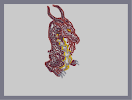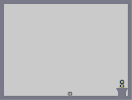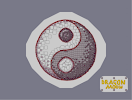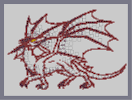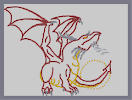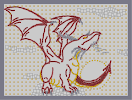New style dragon The story of a small plant 3D Yin-Yang Nam dragon Afternoon Dragon Step 1 Afternoondragon Step02

Pages: (0)

it's going well, keep it up. 4

### topolord,

doesnt that big heavy dragon need strong and muscular wings to lift him,hmmmmmmmmmmmm?

### this WoW

is good, but it its not as helpful as ur comments. I put it in my brain. think i have a lot of time left in my life ;)

### ...

why is this rated so high, its not even done!!!!

### lol

just trying to help...
kinda felt the need to say more then, WOW 5/5!! like all these other ppl...

### nemetacyst,

Thanks for ur critic. But i only need training. its a question of time. :)

### Ummm

why are you like perfect at drawing dragons...
I was just wondering...

### dragon

i figured this was the easiest place for me to remind you about it...
im not advertising...most of us have seen it anyway...i know...dont write annoying messages...thank you for your time

### same problem

as in the first one. the wing arms are to large and muscular.
I would also say the neck is a little to large also.
Head looks good, i like the back leg and the clouds especially

### actually

im not sure about the legs...they look a little fat...and placed high...but they arnt bad...maybe i should jsut wait till u finish to critize them...

but the wing...definately strange...

### um...

i suggest you redo the legs...and upper part of the wing muscles...
they look kinda messed up...and the wing on the point of view side doesnt look attached properly...shouldnt it look a little more like a shoulder joint? not just some random thing coming out of it??

dont get me wrong, its not bad...i just think it needs a good deal of work...

### Great

Really good, but ugly when close up (as all n arts are like that) 5/5

### Hmm

The head is a bit odd (Even in thumbnail mode

Just keep working at it! (Maybe making the Rising sun "Red" for Japan)

### I didnt like it much either

maybe the next one?

### wow

amazing, once again. 5/5

### meh

and i'm the one to rate it down again. it doesn't seem much for me. besides, this should be incomplete.

### This Guide Is Great!

Can't Wait Till 2Moz!

Simple Steps Leading To A So Far Great Pic, Keep It Up!

5/5 For Every Part So Far.